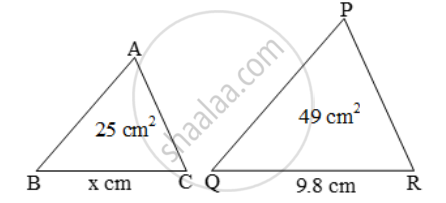# The areas of two similar triangles ∆ABC and ∆PQR are 25 cm2 and 49 cm2 respectively. If QR = 9.8 cm, find BC - Mathematics

Sum

The areas of two similar triangles ∆ABC and ∆PQR are 25 cm2 and 49 cm2 respectively. If QR = 9.8 cm, find BC

#### Solution

It is being given that ∆ABC ~ ∆PQR, ar (∆ABC) = 25 cm2 and ar (∆PQR) = 49 cm2 .

We know that the ratio of the areas of two similar triangles is equal to the ratio of the squares of their corresponding sides.\therefore \text{ }\frac{ar\ (\Delta ABC)}{ar\ (\DeltaPQR)}=\frac{BC^{2}}{QR^{2}}

\Rightarrow \frac{25}{49}=\frac{x^{2}}{(9.8)^{2}}

\Rightarrowx^{2}=( \frac{25}{49}\times 9.8\times 9.8)

\Rightarrow x=\sqrt{\frac{25}{49}\times 9.8\times 9.8}=(5/7xx9.8)=(5xx1.4)=7

Hence BC = 7 cm.

Concept: Areas of Similar Triangles
Is there an error in this question or solution?